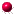XMM-Newton Science Analysis System

eimsim (eimsim-2.4.2) [xmmsas_20211130_0941-20.0.0]

### Attempt to match detected and simulated sources

This function may be performed alone by calling the script with entrystage and finalstage=compare'. The actual processing is done by a task called srccompare.

In order to asses how well the source detection machinery performs, we need some way to (i) match every detection with a unique member of the list of simulated sources which is the most likely identification, and (ii) measure the probablity that the match arose by chance. The obvious answer to the first requirement seems to be to find that simulated source which is nearest' in both position and flux to the detected source. This intuition can be quantified by imagining that both simulated and detected sources are represented by points in an abstract 3-dimensional space in which the first two axes record the source position,and the third records the source flux. Let us define a quantityin this space by the equationwhere,andrepresent position and flux respectively. Thequantities represent the uncertainties which were determined by the source-detection procedure. For each detected source, we define its matching simulated source' as the one which minimizesfor that detection. Let us denote this minimum value ofby. The probability can then be obtained as follows. First, consider the ellipsoidal surface defined byFrom the definition of, this ellipsoid has the following properties:

• It is centred on the position' in this abstract 3-dimensional space of the detected source.
• The principle axes of the ellipse preserve the ratios between the uncertainties. Indeed one can visualize the process of searching for a match as inflating' the ellipsoid as one inflates a balloon, until its edge intersects a simulated source.
• The ellipsoid just touches the matching simulated source.
• No other simulated source is found inside it.

Intuition suggests that the larger the ellipse, or the larger the value of, the less likely it is that the detection is genuine'. Again we quantify this intuition by integrating the probability density distribution of simulated sources in position and flux over the ellipsoidal volume to give, the expectation value for the number of simulated sources which would fall inside the ellipsoid by chance. Ok, we said above that there are zero sim sources within the ellipsoid - but that was in a single, particular case. What we want to test now is the null hypothesis, ie to ask how many simulated sources, on average, we would expect to land inside our ellipsoid if we threw the chips at random.

Having calculated, it is fairly easy to see that the probabilityof the null hypothesis is given by(3)

There is a slight issue here, in that the simulated sources are not evenly distributed in: the number of sources per flux interval increases greatly at low flux. This leads to a bias towards matching with fainter sources. In previous versions of eimsim I assumed that this was a bad thing, and took steps to transform the flux coordinate to correct for this. This is the point of the FLUXRAND business described in section 4.3.1. Now I am no longer sure that this is the case. In real life, we expect the gradient of number density with flux to bias the detected flux - this is called Eddington bias. Maintaining this bias during the matching stage ought to help correct for this. What concerns me more now is that the + and - flux uncertainties ought not to be the same in a simple flux scale: one would expect that the + one ought to be larger. Perhaps then the correct way to transform the flux scale before matching is to take its square root, which should even up the uncertainties. What I have done is provide the facility in eimsim to do any one of three things, namely (i) leave the flux alone; (ii) transform it to the FLUXRAND scale, in which the simulated sources are evenly distributed; (iii) transform the flux scale by taking square roots of flux. Comparison of empirical results ought to show which is the best procedure.

The following additional columns are written to the list of detected sources:

 Name Data type Units Comment X 4-byte real arcsec-coordinate of det source. Y 4-byte real arcsec-coordinate of det source. X_ERR 4-byte real arcsec-coordinate error of det source. Y_ERR 4-byte real arcsec-coordinate error of det source. SIM_X 4-byte real arcsec-coordinate of matching sim source. SIM_Y 4-byte real arcsec-coordinate of matching sim source. SIM_FLUX 4-byte real erg cmsFlux of matching sim source. SIM_INDX 4-byte int From simlist column INDEX. SIM_INV_SENSY 4-byte real From simlist column INV_SENSY. R_SIGMAS 4-byte real. MATCH_PNULL 4-byte realfrom equation 3. SIM_LINF 4-byte real From simlist column FLUXRAND. FLAG 4-byte int

If the user chooses to take the square root of the flux coordinate then the following additional columns are written:

 ROOTF 4-byte real Square root of det source FLUX. ROOTF_ERR 4-byte real The appropriate error in L. SIM_ROOTF 4-byte real Square root of sim source FLUX.

The FLAG column is hardly used at present, but may be found useful in further analysis. Only bit 0 is set by task srccompare. If the same simulated source is claimed' by more than one detected source, bit 0 of the flag column is set for all the claimants except that with the smallest value of MATCH_PNULL.

This section also writes a keyword COMPARED=T' to the table header.

XMM-Newton SOC -- 2021-11-30